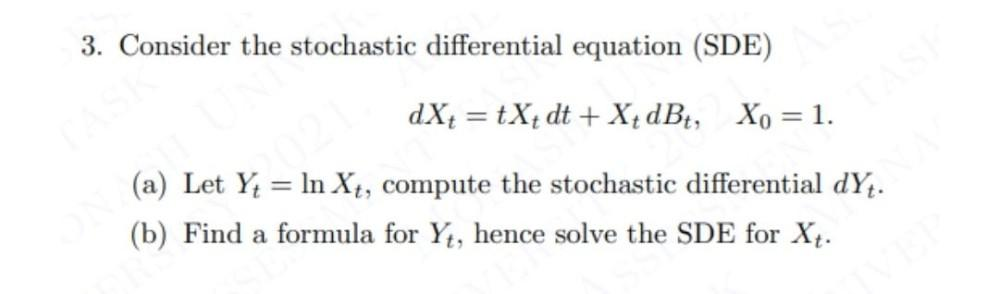Question:

# 3. Consider the stochastic differential equation (SDE) dX= tX+ dt + X{ dBť, Xo = 1. (a) Let Yt = ln Xt, compute the stochastic d3. Consider the stochastic differential equation (SDE) dX= tX+ dt + X{ dBť, Xo = 1. (a) Let Yt = ln Xt, compute the stochastic differential dYt. (b) Find a formula for Yt, hence solve the SDE for Xt.